• Sorting is one of the most used features in programming. And it will take time to complete the sorting if we didn’t use the correct algorithm.

We will walk you through the different sorting algorithms with every step that comes in implementation. The implementation part will be in Python. You can easily convert it into any language once you get the algorithm. That’s the matter of language syntax.

We will see different algorithms from worst to best in this tutorial. So, don’t worry. Follow the article and implement them.

Let’s dive into the sorting algorithms.

## Insertion Sort

Insertion sort is one of the simple sorting algorithms. It’s easy to implement. And it will cost you more time to sort an array. It won’t be used in most of the cases for sorting for larger arrays.

The insertion sort algorithm maintains sorted and unsorted sub-parts in the given array.

The sorted sub-part contains only the first element at the beginning of the sorting process. We will take an element from the unsorted array and place them in the correct position in the sorted sub-array.

Let’s see the visual illustrations of insertion sort step by step with an example.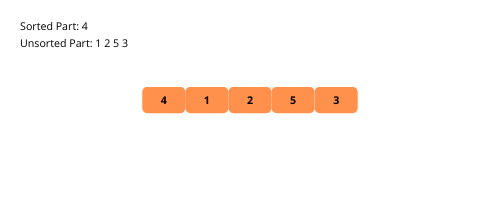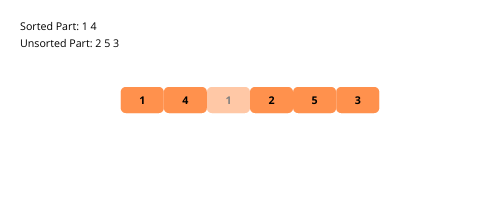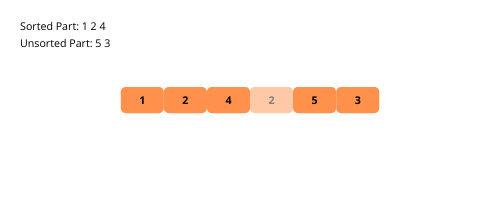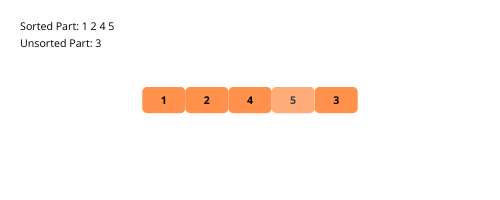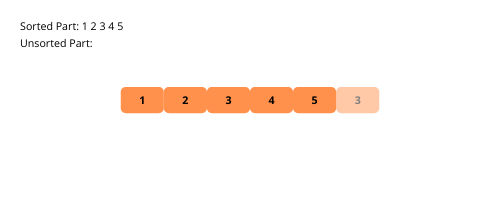Let’s see the steps to implement the insertion sort.

• Initialize the array with dummy data (integers).
• Iterate over the given array from the second element.
• Take the current position and element in two variables.
• Write a loop that iterates until the first element of the array or the element occurs that is less than the current element.
• Update the current element with the previous element.
• Decrement of the current position.
• Here, the loop must reach either the start of the array or find a smaller element than the current element. Replace the current position element with the current element.

The time complexity of the insertion sort is O(n^2), and the space complexity if O(1).

That’s it; we’ve sorted the given array. Let’s run the following code. I hope you have installed Python, if not check out the installation guide. Alternatively, you can use an online Python compiler.

``````def insertion_sort(arr, n):
for i in range(1, n):

## current position and element
current_position = i
current_element = arr[i]

## iteratin until
### it reaches the first element or
### the current element is smaller than the previous element
while current_position > 0 and current_element <
arr[current_position - 1]:
## updating the current element with previous element
arr[current_position] = arr[current_position - 1]

## moving to the previous position
current_position -= 1

## updating the current position element
arr[current_position] = current_element

if __name__ == '__main__':
## array initialization
arr = [3, 4, 7, 8, 1, 9, 5, 2, 6]
insertion_sort(arr, 9)

## printing the array
print(str(arr))``````

## Selection Sort

The selection sort is similar to the insertion sort with a slight difference. This algorithm also divides the array into sorted and unsorted sub-parts. And then, on each iteration, we will take the minimum element from the unsorted sub-part and place it in the last position of the sorted sub-part.

Let’s illustrations of selection sort for better understanding.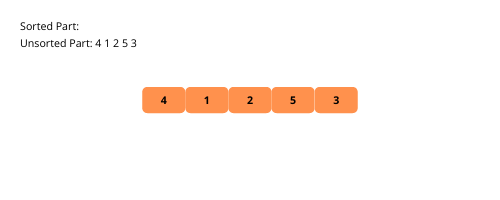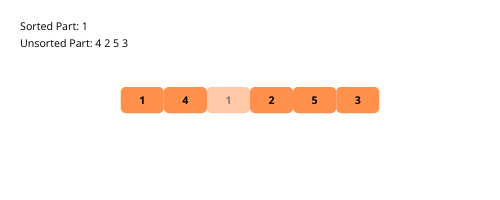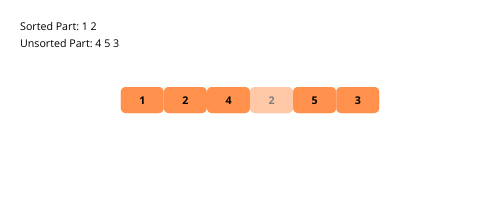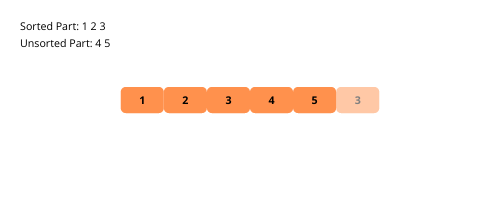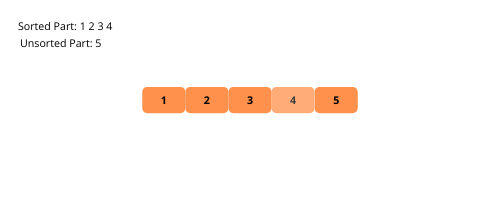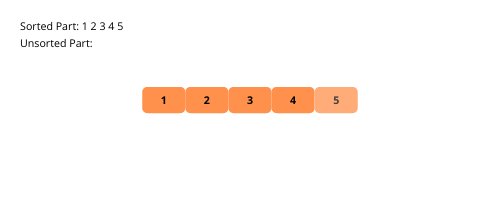Let’s see the steps to implement the selection sort.

• Initialize the array with dummy data (integers).
• Iterate over the given array.
• Maintain the index of the minimum element.
• Write a loop that iterates from the current element to the last element.
• Check whether the current element is less than the minimum element or not.
• If the current element less than the minimum element, then replace the index.
• We have the minimum element index with us. Swap the current element with the minimum element using the indexes.

The time complexity of the selection sort is O(n^2), and the space complexity if O(1).

Try to implement the algorithm as it is similar to the insertion sort. You can see the code below.

``````def selection_sort(arr, n):
for i in range(n):

## to store the index of the minimum element
min_element_index = i
for j in range(i + 1, n):
## checking and replacing the minimum element index
if arr[j] < arr[min_element_index]:
min_element_index = j

## swaping the current element with minimum element
arr[i], arr[min_element_index] = arr[min_element_index], arr[i]

if __name__ == '__main__':
## array initialization
arr = [3, 4, 7, 8, 1, 9, 5, 2, 6]
selection_sort(arr, 9)

## printing the array
print(str(arr))``````

## Bubble Sort

Bubble sort is a simple algorithm. It swaps the adjacent elements on each iteration repeatedly until the given array is sorted.

It iterates over the array and moves the current element to the next position until it is less than the next element.

Illustrations help us understand the bubble sort visually. Let’s see them.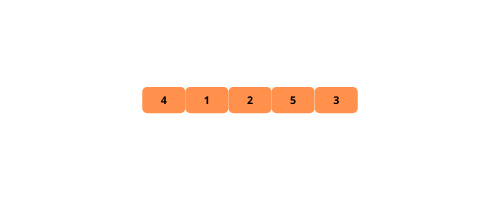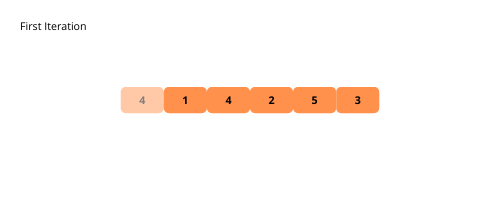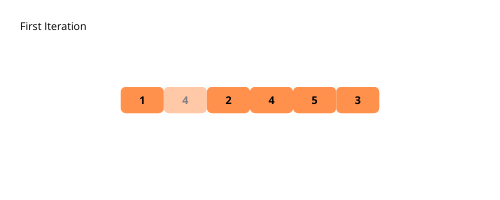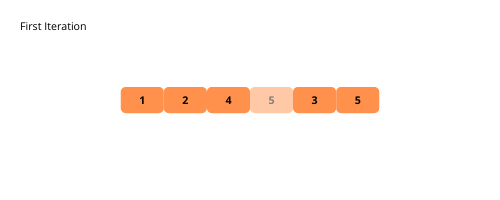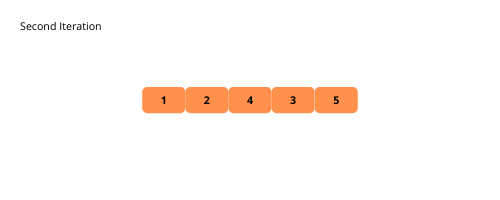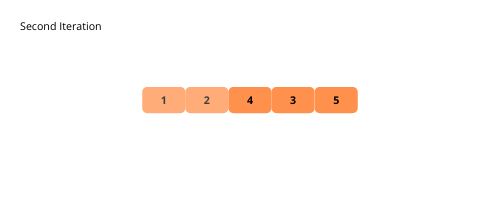Let’s see the steps to implement the bubble sort.

1. Initialize the array with dummy data (integers).
2. Iterate over the given array.
1. Iterate from to n-i-1. The last i elements are already sorted.
1. Check whether the current element is greater than the next element or not.
2. If the current element is greater than the next element, then swap the two elements.

The time complexity of the bubble sort is O(n^2), and the space complexity if O(1).

You can easily implement the bubble sort by now. Let’s see the code.

``````def bubble_sort(arr, n):
for i in range(n):
## iterating from 0 to n-i-1 as last i elements are already sorted
for j in range(n - i - 1):
## checking the next element
if arr[j] > arr[j + 1]:
arr[j], arr[j + 1] = arr[j + 1], arr[j]

if __name__ == '__main__':
## array initialization
arr = [3, 4, 7, 8, 1, 9, 5, 2, 6]
bubble_sort(arr, 9)

## printing the array
print(str(arr))``````

## Merge Sort

Merge sort is a recursive algorithm to sort the given array. It’s more efficient than the previously discussed algorithms in terms of time complexity. It follows the divide and conquers approach.

The merge sort algorithm divides the array into two halves and sorts them separately. After sorting the two halves of the array, it merges them into a single sorted array.

As it’s a recursive algorithm, it divides the array till the array becomes the simplest (array with one element) one to sort.

It’s time for illustration. Let’s see it.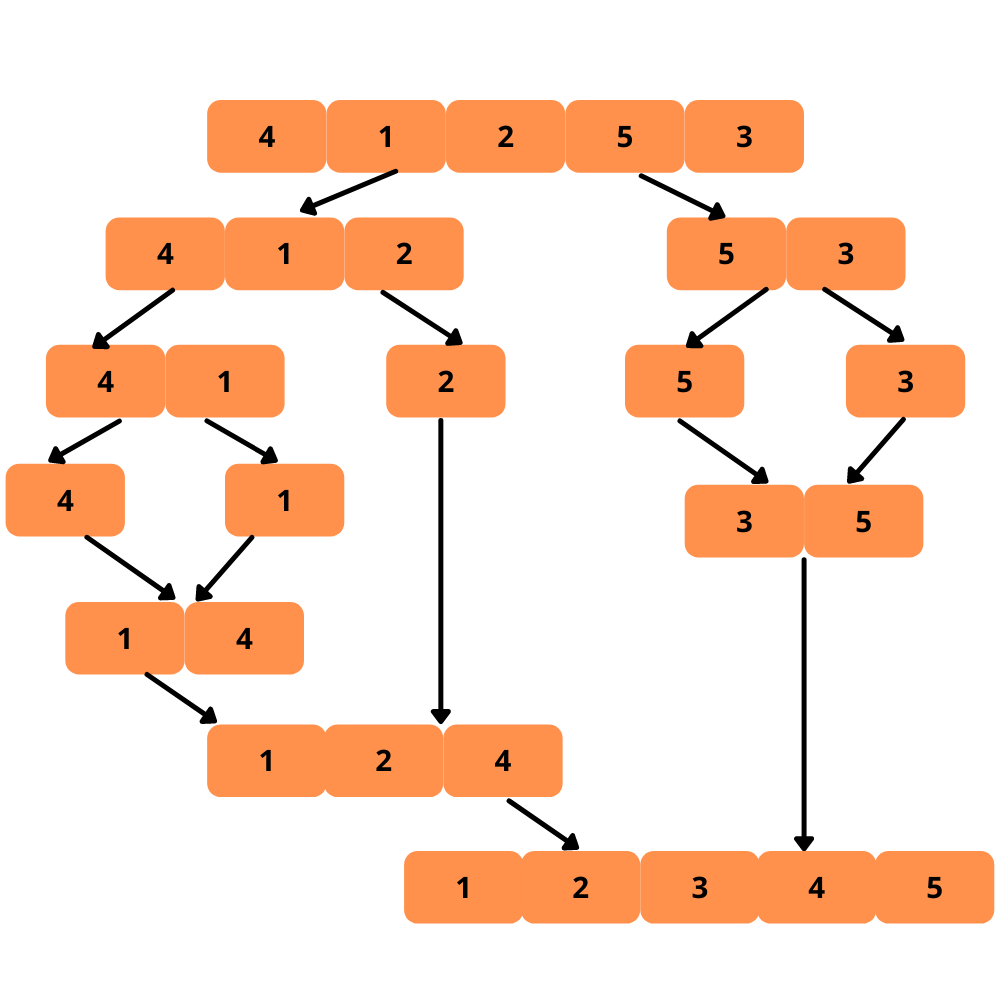Let’s see the steps to implement the merge sort.

• Initialize the array with dummy data (integers).
• Write a function called merge to merge sub-arrays into a single sorted array. It accepts the arguments array, left, mid, and right indexes.
• Get the lengths of left and right sub-arrays lengths using the given indexes.
• Copy the elements from the array into the respective left and right arrays.
• Iterate over the two sub-arrays.
• Compare the two sub-arrays elements.
• Replace the array element with the smaller element from the two sub-arrays for sorting.
• Check whether any elements are remaining in both the sub-arrays.
• Add them to the array.
• Write a function called merge_sort with parameters array, left and right indexes.
• If the left index is greater than or equal to the right index, then return.
• Find the middle point of the array to divide the array into two halves.
• Recursively call the merge_sort using the left, right, and mid indexes.
• After the recursive calls, merge the array with the merge function.

The time complexity of the merge sort is O(nlogn), and the space complexity if O(1).

That’s it for the merge sort algorithm implementation. Check the code below.

``````def merge(arr, left_index, mid_index, right_index):
## left and right arrays
left_array = arr[left_index:mid_index+1]
right_array = arr[mid_index+1:right_index+1]

## getting the left and right array lengths
left_array_length = mid_index - left_index + 1
right_array_length = right_index - mid_index

## indexes for merging two arrays
i = j = 0

## index for array elements replacement
k = left_index

## iterating over the two sub-arrays
while i < left_array_length and j < right_array_length:

## comapring the elements from left and right arrays
if left_array[i] < right_array[j]:
arr[k] = left_array[i]
i += 1
else:
arr[k] = right_array[j]
j += 1
k += 1

## adding remaining elements from the left and right arrays
while i < left_array_length:
arr[k] = left_array[i]
i += 1
k += 1

while j < right_array_length:
j += 1
k += 1

def merge_sort(arr, left_index, right_index):
## base case for recursive function
if left_index >= right_index:
return

## finding the middle index
mid_index = (left_index + right_index) // 2

## recursive calls
merge_sort(arr, left_index, mid_index)
merge_sort(arr, mid_index + 1, right_index)

## merging the two sub-arrays
merge(arr, left_index, mid_index, right_index)

if __name__ == '__main__':
## array initialization
arr = [3, 4, 7, 8, 1, 9, 5, 2, 6]
merge_sort(arr, 0, 8)

## printing the array
print(str(arr))``````

### Conclusion

There are many other sorting algorithms, but above are some of the frequently used. Hope you enjoyed learning the sorting.

Next, find out about search algorithms.

Happy Coding 🙂 👨‍💻